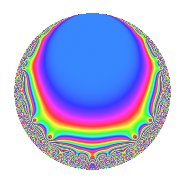# Properties

 Label 12.5.dLevel 12 Weight 5 Character orbit d Rep. character $$\chi_{12}(7,\cdot)$$ Character field $$\Q$$ Dimension 4 Newforms 1 Sturm bound 10 Trace bound 0

# Related objects

## Defining parameters

 Level: $$N$$ = $$12 = 2^{2} \cdot 3$$ Weight: $$k$$ = $$5$$ Character orbit: $$[\chi]$$ = 12.d (of order $$2$$ and degree $$1$$) Character conductor: $$\operatorname{cond}(\chi)$$ = $$4$$ Character field: $$\Q$$ Newforms: $$1$$ Sturm bound: $$10$$ Trace bound: $$0$$

## Dimensions

The following table gives the dimensions of various subspaces of $$M_{5}(12, [\chi])$$.

Total New Old
Modular forms 10 4 6
Cusp forms 6 4 2
Eisenstein series 4 0 4

## Trace form

 $$4q$$ $$\mathstrut +\mathstrut 6q^{2}$$ $$\mathstrut -\mathstrut 20q^{4}$$ $$\mathstrut +\mathstrut 24q^{5}$$ $$\mathstrut -\mathstrut 18q^{6}$$ $$\mathstrut -\mathstrut 108q^{9}$$ $$\mathstrut +\mathstrut O(q^{10})$$ $$4q$$ $$\mathstrut +\mathstrut 6q^{2}$$ $$\mathstrut -\mathstrut 20q^{4}$$ $$\mathstrut +\mathstrut 24q^{5}$$ $$\mathstrut -\mathstrut 18q^{6}$$ $$\mathstrut -\mathstrut 108q^{9}$$ $$\mathstrut -\mathstrut 172q^{10}$$ $$\mathstrut +\mathstrut 180q^{12}$$ $$\mathstrut +\mathstrut 296q^{13}$$ $$\mathstrut +\mathstrut 600q^{14}$$ $$\mathstrut +\mathstrut 112q^{16}$$ $$\mathstrut -\mathstrut 600q^{17}$$ $$\mathstrut -\mathstrut 162q^{18}$$ $$\mathstrut -\mathstrut 1368q^{20}$$ $$\mathstrut -\mathstrut 144q^{21}$$ $$\mathstrut -\mathstrut 1128q^{22}$$ $$\mathstrut +\mathstrut 1296q^{24}$$ $$\mathstrut +\mathstrut 972q^{25}$$ $$\mathstrut +\mathstrut 1692q^{26}$$ $$\mathstrut +\mathstrut 1488q^{28}$$ $$\mathstrut +\mathstrut 888q^{29}$$ $$\mathstrut -\mathstrut 1980q^{30}$$ $$\mathstrut -\mathstrut 2784q^{32}$$ $$\mathstrut +\mathstrut 720q^{33}$$ $$\mathstrut -\mathstrut 484q^{34}$$ $$\mathstrut +\mathstrut 540q^{36}$$ $$\mathstrut -\mathstrut 4408q^{37}$$ $$\mathstrut +\mathstrut 4680q^{38}$$ $$\mathstrut +\mathstrut 1664q^{40}$$ $$\mathstrut +\mathstrut 552q^{41}$$ $$\mathstrut -\mathstrut 2088q^{42}$$ $$\mathstrut -\mathstrut 3696q^{44}$$ $$\mathstrut -\mathstrut 648q^{45}$$ $$\mathstrut -\mathstrut 384q^{46}$$ $$\mathstrut +\mathstrut 1008q^{48}$$ $$\mathstrut -\mathstrut 572q^{49}$$ $$\mathstrut -\mathstrut 1038q^{50}$$ $$\mathstrut +\mathstrut 6008q^{52}$$ $$\mathstrut +\mathstrut 5112q^{53}$$ $$\mathstrut +\mathstrut 486q^{54}$$ $$\mathstrut +\mathstrut 1728q^{56}$$ $$\mathstrut +\mathstrut 5616q^{57}$$ $$\mathstrut -\mathstrut 124q^{58}$$ $$\mathstrut -\mathstrut 2664q^{60}$$ $$\mathstrut +\mathstrut 4232q^{61}$$ $$\mathstrut -\mathstrut 7224q^{62}$$ $$\mathstrut -\mathstrut 14720q^{64}$$ $$\mathstrut -\mathstrut 18192q^{65}$$ $$\mathstrut +\mathstrut 4824q^{66}$$ $$\mathstrut +\mathstrut 5496q^{68}$$ $$\mathstrut -\mathstrut 9792q^{69}$$ $$\mathstrut +\mathstrut 6096q^{70}$$ $$\mathstrut +\mathstrut 8840q^{73}$$ $$\mathstrut -\mathstrut 4116q^{74}$$ $$\mathstrut -\mathstrut 1872q^{76}$$ $$\mathstrut +\mathstrut 20928q^{77}$$ $$\mathstrut +\mathstrut 9900q^{78}$$ $$\mathstrut +\mathstrut 25632q^{80}$$ $$\mathstrut +\mathstrut 2916q^{81}$$ $$\mathstrut +\mathstrut 3740q^{82}$$ $$\mathstrut -\mathstrut 10512q^{84}$$ $$\mathstrut -\mathstrut 10256q^{85}$$ $$\mathstrut -\mathstrut 19560q^{86}$$ $$\mathstrut -\mathstrut 8640q^{88}$$ $$\mathstrut -\mathstrut 25080q^{89}$$ $$\mathstrut +\mathstrut 4644q^{90}$$ $$\mathstrut +\mathstrut 18816q^{92}$$ $$\mathstrut -\mathstrut 17136q^{93}$$ $$\mathstrut -\mathstrut 5232q^{94}$$ $$\mathstrut -\mathstrut 8352q^{96}$$ $$\mathstrut +\mathstrut 23048q^{97}$$ $$\mathstrut -\mathstrut 5850q^{98}$$ $$\mathstrut +\mathstrut O(q^{100})$$

## Decomposition of $$S_{5}^{\mathrm{new}}(12, [\chi])$$ into irreducible Hecke orbits

Label Dim. $$A$$ Field CM Traces $q$-expansion
$$a_2$$ $$a_3$$ $$a_5$$ $$a_7$$
12.5.d.a $$4$$ $$1.240$$ $$\Q(\sqrt{-3}, \sqrt{13})$$ None $$6$$ $$0$$ $$24$$ $$0$$ $$q+(2-\beta _{1})q^{2}-\beta _{2}q^{3}+(-4-3\beta _{1}+\cdots)q^{4}+\cdots$$

## Decomposition of $$S_{5}^{\mathrm{old}}(12, [\chi])$$ into lower level spaces

$$S_{5}^{\mathrm{old}}(12, [\chi]) \cong$$ $$S_{5}^{\mathrm{new}}(4, [\chi])$$$$^{\oplus 2}$$